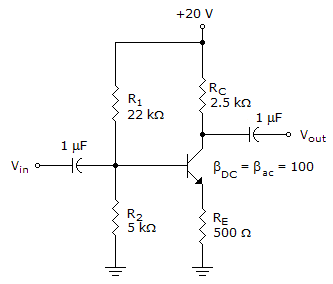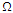# Electronic Devices - BJT Amplifiers

### Exercise :: BJT Amplifiers - General Questions

21.

Refer to this figure. Calculate the value of Rin(tot).A. 37.7 kB. 3.77 kC. 378D. 2.25 kExplanation:

No answer description available for this question. Let us discuss.

22.

What is the range of the current gain for BJT transistor amplifiers?

 A. less than 1 B. 1 to 100 C. above 100 D. All of the above

Explanation:

No answer description available for this question. Let us discuss.

23.

What does the negative sign in the voltage gain of the common-emitter fixed-bias configuration indicate?

 A. The output and input voltages are 180º out of phase. B. Gain is smaller than 1. C. Gain is larger than 1. D. None of the above

Explanation:

No answer description available for this question. Let us discuss.

24.

For the common-emitter fixed-bias configuration, there is a ________ phase shift between the input and output signals.

 A. 0º B. 45º C. 90º D. 180º

Explanation:

No answer description available for this question. Let us discuss.

25.

Which one of the following configurations has the lowest input impedance?

 A. Fixed-bias B. Common-base C. Emitter-follower D. Voltage-divider?